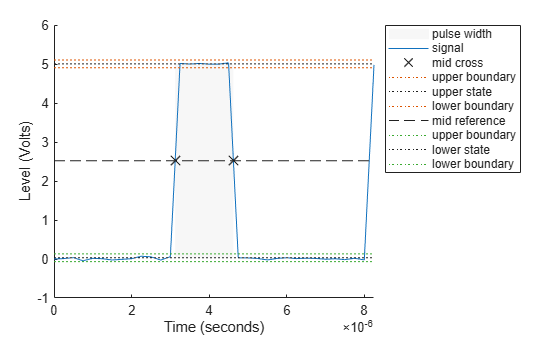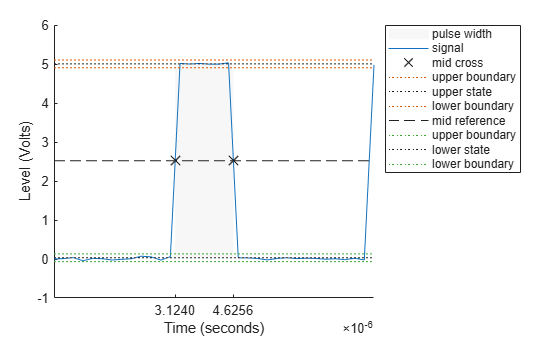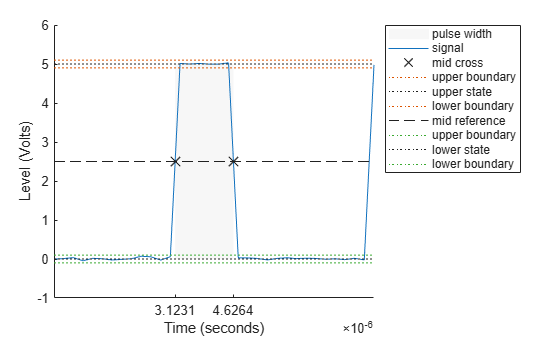# pulsewidth

Bilevel waveform pulse width

## Syntax

w = pulsewidth(x)
w = pulsewidth(x,fs)
w = pulsewidth(x,t)
[w,initcross] = pulsewidth(___)
[w,initcross,finalcross] = pulsewidth(___)
[w,initcross,finalcross,midlev] = pulsewidth(___)
W = pulsewidth(___,Name,Value)
pulsewidth(___)

## Description

w = pulsewidth(x) returns the time differences between the midreference level instants of the initial and final transitions of each positive-polarity pulse in the input bilevel waveform.
w = pulsewidth(x,fs) specifies the sample rate fs in hertz. The first sample in the waveform corresponds to t = 0.

example

w = pulsewidth(x,t) specifies the sample instants t.
[w,initcross] = pulsewidth(___) returns initcross, the midreference level instants of the initial transition of each pulse. You can specify an input combination from any of the previous syntaxes.

example

[w,initcross,finalcross] = pulsewidth(___) returns finalcross, the midreference level instants of the final transition of each pulse.
[w,initcross,finalcross,midlev] = pulsewidth(___) returns the waveform value midlev that corresponds to the midreference level.

example

W = pulsewidth(___,Name,Value) specifies additional options using one or more Name,Value arguments.
pulsewidth(___) plots the signal and darkens the regions of each pulse where the function computes the pulse width. The function marks the location of the midcrossings and their associated reference level. The function also plots the state levels and their associated lower and upper boundaries.

## Examples

collapse all

Compute the pulse width of a bilevel waveform sampled at 4 MHz.

load('pulseex.mat','x','t') w = pulsewidth(x,t)
w = 1.5016e-06 

Plot the waveform and annotate the pulse width.

pulsewidth(x,t);Compute the initial and final transition occurrences for a bilevel waveform sampled at 4 MHz.

load('pulseex.mat','x','t'); fs = 4e6; [w,initcross,finalcross] = pulsewidth(x,fs);

Plot the result, annotated with the transition occurrences.

pulsewidth(x,fs); ax = gca; ax.XTick = [initcross finalcross];Specify the state levels for the bilevel waveform instead of estimating the levels from the data. Specify the low-state level as 0 and the high-state level as 5.

load('pulseex.mat','x','t') fs = 4e6; [w,initcross,finalcross] = pulsewidth(x,fs,'StateLevels',[0 5]);

Plot the result annotated with the transition occurrences.

pulsewidth(x,fs,'StateLevels',[0 5]); ax = gca; ax.XTick = [initcross finalcross];## Input Arguments

collapse all

Bilevel waveform, specified as a real-valued vector.

Sample rate in hertz, specified as a real positive scalar.

Sample instants, specified as a vector. The length of T must equal the length of the bilevel waveform x.

### Name-Value Arguments

Specify optional pairs of arguments as Name1=Value1,...,NameN=ValueN, where Name is the argument name and Value is the corresponding value. Name-value arguments must appear after other arguments, but the order of the pairs does not matter.

Before R2021a, use commas to separate each name and value, and enclose Name in quotes.

Example: 'StateLevels',[0 5] specifies a low-state level of 0 and a high-state level of 5.

Midreference level as a percentage of the waveform amplitude, specified as a real-valued scalar. For more information, see Midreference Level.

Pulse polarity, specified as 'positive' or 'negative'. If you specify 'positive', the function looks for pulses with positive-going (positive polarity) initial transitions. If you specify 'negative', the pulsewidth function looks for pulses with negative-going (negative polarity) initial transitions. For more information, see Pulse Polarity.

Low- and high-state levels, specified as a 1-by-2 real-valued vector. The first element is the low-state level, and the second element is the high-state level. If you do not specify low- and high-state levels, the pulsewidth function estimates the state levels from the input waveform using the histogram method. For a detailed description of the histogram method, see State-Level Estimation.

Tolerance levels (lower- and upper-state boundaries) expressed as a percentage, specified as a real-valued scalar. For more information, see State-Level Tolerances.

## Output Arguments

collapse all

Pulse widths in seconds, returned as a vector. The pulse width is the time difference between the initial and final transitions of a pulse. The times of the initial and final transitions are referred to as transition occurrence instants in .

Note

Because the pulsewidth function uses interpolation to determine the midreference level instants, w might contain values that do not correspond to sampling instants of the bilevel waveform x.

Midreference level instants of the initial transition of each pulse, returned as a column vector.

Midreference level instants of the final transition of each pulse, returned as a column vector.

Waveform value corresponding to the midreference level, returned as a scalar.

collapse all

### State-Level Estimation

To determine the transitions, the pulsewidth function estimates the low- and high-state levels of input x by using a histogram method with these steps.

1. Determine the minimum and maximum amplitudes of the data.

2. For the specified number of histogram bins, determine the bin width, which is the ratio of the amplitude range to the number of bins.

3. Sort the data values into the histogram bins.

4. Identify the lowest and highest indexed histogram bins with nonzero counts.

5. Divide the histogram into two subhistograms.

6. Compute the state levels by determining the mode or mean of the upper and lower histograms.

The function identifies all regions that cross the upper-state boundary of the low state and the lower-state boundary of the high state. The low-state and high-state boundaries are expressed as the state level plus or minus a multiple of the difference between the state levels.

### Midreference Level

The midreference level in a bilevel waveform with low-state level, S1, and high-state level, S2, is

${S}_{1}+\frac{1}{2}\left({S}_{2}-{S}_{1}\right)$

### Midreference Level Instant

The midreference level instant is

${t}_{50%}={t}_{50%}+\left(\frac{{t}_{50{%}_{+}}-{t}_{50{%}_{-}}}{{y}_{50{%}_{+}}-{y}_{50{%}_{-}}}\right)\left({y}_{50{%}_{+}}-{y}_{50{%}_{-}}\right)$

where:

• y50% denotes the midreference level.

• t50%- and t50%+ denote the two consecutive sampling instants corresponding to the waveform values that are nearest in value to y50%.

• y50%- and y50%+ denote the waveform values at t50%- and t50%+.

### Pulse Polarity

If the pulse has a positive-going initial transition, the pulse has positive polarity. Equivalently, a positive-polarity (positive-going) pulse has a terminating state that is more positive than the originating state. This figure shows a positive-polarity pulse.If the pulse has a negative-going initial transition, the pulse has negative polarity. Equivalently, a negative-polarity (negative-going) pulse has an originating state that is more positive than the terminating state. This figure shows a negative-polarity pulse.### State-Level Tolerances

You can specify lower- and upper-state boundaries for each state level. Define the boundaries as the state level plus or minus a scalar multiple of the difference between the high state and the low state. To provide a useful tolerance region, specify the scalar as a small number such as 2/100 or 3/100. In general, theregion for the low state is defined aswhereis the low-state level andis the high-state level. Replace the first term in the equation withto obtain thetolerance region for the high state.

This figure shows lower and upper 5% state boundaries (tolerance regions) for a positive-polarity bilevel waveform. The thick dashed lines indicate the estimated state levels.IEEE Standard 181. IEEE® Standard on Transitions, Pulses, and Related Waveforms (2003).